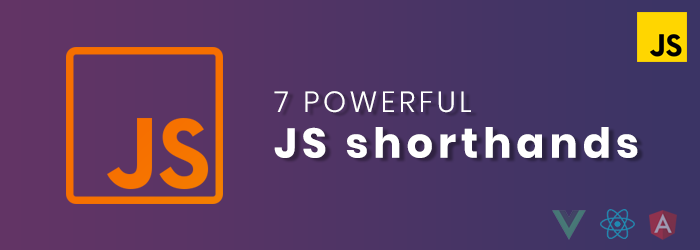# Technology Blog

Look deep into latest news and innovations happening in the Tech industry with our highly informational blog.

# 7 useful JavaScript shorthands you must know about

JavaScript is a very powerful programming language these days. There is a lot that you can do with it. In addition to that, JavaScript has a huge ecosystem that allows you to discover a lot of useful tools, frameworks, and libraries.JavaScript has a lot of powerful features and tools that make the life of the developer much easier. The syntax contains a lot of shorthands that you can use to write JavaScript code much faster and reduce lines. Especially in the latest ECMAScript specifications.

In this article, we will give you a list of shorthands that you can use in JavaScript. So let’s get right into it.

### 1. Convert string to a number

Normally, to convert a string to a number, we use the method parseInt() . However, there is a shorthand that allows us to do that.

By using the unary operator + , we can easily convert a string into a number.

Here is an example:

```let speed = "60";

console.log(typeof speed); //string

console.log(typeof +speed); //number

console.log(+speed); //60, not "60".```

As you can see, only by adding + at the beginning of the variable speed , we were able to convert the speed to a number.

There is also another shorthand to convert from a number into a string. It’s by simply adding an empty string to the number.

Here is an example:

```let speed = 100;

speed += ""; //returns "100" , not 100.

console.log(typeof speed); //string```

### 2. Using the ternary operator

Another shorthand is the ternary operator, it allows us to easily write conditional statements in a short way using the keywords ? and : .

Here is an example using IF else statements:

Longhand:

```let speed = 80;

if(speed < 30){

console.log('slow');

}else if(speed > 60){

console.log('fast');

}else{

console.log('between 30 and 60');

}

//output: fast
```

Here is the same example, but now using the ternary operator instead:

Shorthand:

```let speed = 80;

console.log(speed < 30 ? 'slow': speed > 60 ? 'fast'
: 'between 30 & 60');

//output: fast```

As you can see, by using the ternary operator shorthand, we were able to easily reduce the amount of code we had to write. It only took us 2 lines of code to write the conditional statements.

### 3. Short-circuit evaluation

Have a look at the below example using an IF statement.

Longhand:

```let isOnline = true;

if(isOnline){
console.log("Online");
}

//returns "online"```

We can write the same statement using the short circuit && . Here is the example:

Shorthand:

```let isOnline = true;

isOnline && console.log("Online"); //returns "online"```

The short circuit evaluation && is one of the shorthands that allow you to write short conditionals. It’s used between two values, if the first value is truthy, it returns the second value. Otherwise, it returns the first value.

### 4. Flattening an array

The best shorthand to flatten a multidimensional array is by using the method flat(). We have seen, a lot of developers use the method filter, concat, and all other long ways to flatten an array. Maybe because they don’t know about the flat method yet.

So this amazing method allows you to flatten an array in one single line of code. It accepts one optional argument(number), which is the level of flattening(how deep you want to flatten an array).

Here is an example:

```let numbers = [9, [102, 5], [6, 3], 2];

numbers.flat(); //returns [9, 102, 5, 6, 3, 2]```

### 5. Merging and cloning arrays

When it comes to merging and cloning arrays in JavaScript, the spread operator {…} is the best shorthand you can use.

Merging arrays:

```const employees = ["Chris", "John"];

const entrepreneurs = ["James", "Anna"];

//merging both arrays to a new array.

const all = [...employees, ...entrepreneurs];

console.log(all); //output: ["Chris", "John", "James", "Anna"]```

Cloning an array:

```const numbers = [4, 8, 9, 0];

//cloning the numbers array.

const clone = [...numbers];

console.log(clone); //output: [4, 8, 9, 0]```

### 6. For loop shorthand

Mostly when we want to loop through an array using a for loop, we do it like this:

Longhand:

```const users = ["John", "Chris", "Mehdi", "James"];

for(let i = 0; i < users.length; i++){
console.log(users[i]);
}

/*output:
John
Chris
Mehdi
James
*/```

But we can achieve the same thing using the loop for of shorthand:

Shorthand:

```const users = ["John", "Chris", "Mehdi", "James"];

for (let user of users){
console.log(user);
}

/*output:
John
Chris
Mehdi
James*/```

### 7. Arrow functions

Arrow functions are a shorthand that allows you to easily write functions in a short way and reduce your code.

Here are the examples:

Normal function:

```function addNums(x, y){
return x + y;
}

Arrow function:

```const addNums = (x, y)=> x + y;